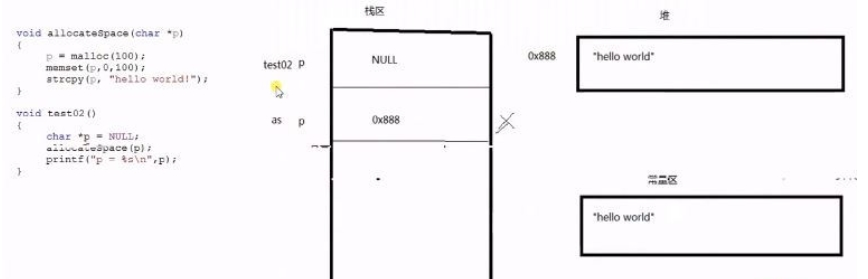C语言深度剖析之查漏补缺

C语言深度剖析之查漏补缺，进行了总结。转载请注明链接，有问题请及时联系博主：Alliswell_WP

int  a;
int  *p = &a;

1) const int*p=&a；

2) int*const p=&a；

3) const int*const p=&a；

# include <stdio.h>
int main(void)
{
int a = 10;
const int *p = &a;
int * q = &a;
*q = 20;
printf("a = %d\n", a);
a = 30;
printf("a = %d\n", a);
//*p = 30;  //这么写就是错的
return 0;
}

a = 20
a = 30

#include <stdio.h>
#include <stdlib.h>
#include <string.h>

int main(void)
{
//const修饰一个变量为只读
const int a = 10;
//a = 100; //err

//指针变量， 指针指向的内存， 2个不同概念
char buf[] = "aklgjdlsgjlkds";

//从左往右看，跳过类型，看修饰哪个字符
//如果是*， 说明指针指向的内存不能改变
//如果是指针变量，说明指针的指向不能改变，指针的值不能修改
const char *p = buf;
// 等价于上面 char const *p1 = buf;
//p = '2'; //err
p = "agdlsjaglkdsajgl"; //ok

char * const p2 = buf;
p2 = '3';
//p2 = "salkjgldsjaglk"; //err

//p3为只读，指向不能变，指向的内存也不能变
const char * const p3 = buf;

return 0;
}

//结构体类型的定义
struct stu
{
char name;
int age;
};

void fun1(struct stu * const p)
{
//p = NULL; //err
p->age = 10; //ok
}

//void fun2(struct stu const*  p)
void fun2(const struct stu *  p)
{
p = NULL; //ok
//p->age = 10; //err
}

void fun3(const struct stu * const p)
{
//p = NULL; //err
//p->age = 10; //err
}

1）含义上的区别
char str[] 是定义一个字符串数组，数组对应的是一块内存区域，而char *str 是定义一个指向字符串的指针，即指向一块内存区域。

#include<stdio.h>
#include<string.h>
int main()
{
char str1[] = "Hello";
char str2[] = "World";
strcpy(str1,str2);
printf("%s\n",str1);
return 0;
}

#include<stdio.h>
#include<string.h>
int main()
{
char *str1 = "Hello";
char *str2 = "World";
strcpy(str1,str2);
printf("%s\n",str1);
return 0;
}

》扩展：

char str[]="hello";

char *foo()
{
char str[]="hello";
return str;
}

#include<stdio.h>
#include<string.h>
char* foo()
{
char *str="hello";
return str;
}
int main()
{
char *q;
q=foo();
printf("q=%s\n",q);
}

2）计算内存的区别

#include <stdio.h>
#include <stdlib.h>
#include <string.h>

int inverse(char *p)
{
if (p == NULL)
{
return -1;
}
char *str = p;
int begin = 0;
int end = strlen(str) - 1;
char tmp;

while (begin < end)
{
//交换元素
tmp = str[begin];
str[begin] = str[end];
str[end] = tmp;

begin++;  //往右移动位置
end--;        //往左移动位置
}

return 0;
}

int main(void)
{
//char *str = "abcdefg"; //文件常量区，内容不允许修改
char str[] = "abcdef";

int ret = inverse(str);
if (ret != 0)
{
return ret;
}

printf("str ========== %s\n", str);
return 0;
}

1）通过返回值分配内存

int *getspace()
{
//malloc函数的返回值是分配区域的起始地址
//函数原型为void *malloc(unsigned int size)；
//其作用是在内存的动态存储区中分配一个长度为size的连续空间
int *p = (int *)malloc(sizeof(int) * 5);//在堆上动态分配内存
if (NULL == p)
{
return NULL;
}
//往分配的内存里面写值
for (int i = 0; i < 5; ++i)//++i 比i++ 的效率高
{
//只要是连续的空间都能使用下标的方式访问
p[i] = 100 + i;
}
return p;

}
void  test()
{
int *ret = getspace();
for (int i = 0; i < 5; ++i)
{
cout << ret[i] << endl;
}

free(ret);//释放堆上动态分配的内存
ret = NULL;//避免出现野指针

}

2）通过参数传递指针分配内存

（1）错误写法

void allocateSpace(char *p)
{
p= (char *)malloc(100);
memset(p, 0, 100);
strcpy(p,"hello world");
}
void  test1()
{
char *ret = NULL;
allocateSpace(ret);
cout << "ret=" << ret;
}（2）正确的写法

void allocateSpace(char **p)
{
char *temp = (char *)malloc(100);
//memset() 函数可新申请的内存进行初始化工作
//将指针变量 p 所指向的前 100 字节的内存单元用一个“整数” 0 替换
memset(temp, 0, 100);
strcpy(temp,"hello world");
*p = temp;//*p=ret，所以实际ret指向malloc分配的内存空间
}
void  test12()
{
char *ret = NULL;
allocateSpace(&ret);
cout << "ret=" << ret;//结果ret=hello world
}

int main() {
struct student{
char c;
}s1;
s1.c="china";
printf("%s",s1.c);
return 0;
}

C语言只有在定义字符数组的时候才能用“=”来初始化变量，其它情况下是不能直接用“=”来为字符数组赋值的，要为字符数组赋值可以用string.h头文件中的strcpy函数来完成。

char a = "123"; /*正确，在定义的时候初始化*/
char a;
a = "123"; /*错误，不能用“=”直接为字符数组赋值*/
strcpy(a, "123"); /*正确，使用strcpy函数复制字符串*/

（1）在声明结构体变量时赋值：

//#include "stdafx.h"//If the vc++6.0, with this line.
#include "stdio.h"
struct stu{
int x;
char name;
};

int main(void){
struct stu s={8,"123"};//这样初始化
printf("%d %s\n",s.x,s.name);
return 0;
}

（2）向数组直接拷贝字符串：

//#include "stdafx.h"//If the vc++6.0, with this line.
#include "stdio.h"
#include "string.h"
struct stu{
int x;
char name;
};

int main(void){
struct stu s;
strcpy(s.name,"abcd");//向name拷贝字符串
s.x=128;
printf("%d %s\n",s.x,s.name);
return 0;
}

(1)首先，其实是忘记了C++的基础问题，C++里面只要涉及char都不能直接通过“=”来赋值，因为C++里面没有提供这个功能。必须使用str开头的函数。只有后来的CString重载来“-，+，=”之后才可以怎么方便的使用。CString str；str=“sasa";.
(2)其次，stu.name="hello";//报错为什么？name是一个10大小的内存空间，而”hello“是一个常量匿名字符串的地址，现在你应该明白了.

C语言深度剖析之查漏补缺，进行了总结。转载请注明链接，有问题请及时联系博主：Alliswell_WP

posted on 2020-09-09 19:05  Alliswell_WP  阅读(143)  评论(0编辑  收藏  举报